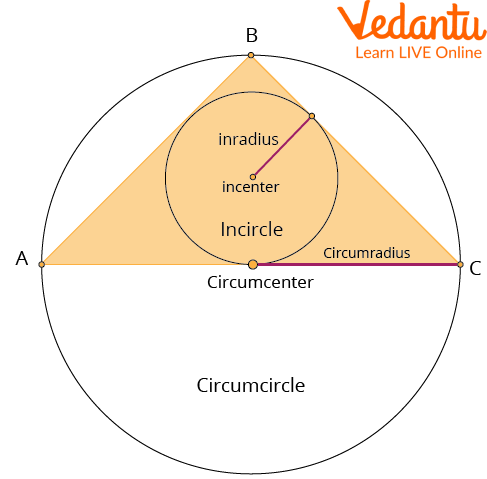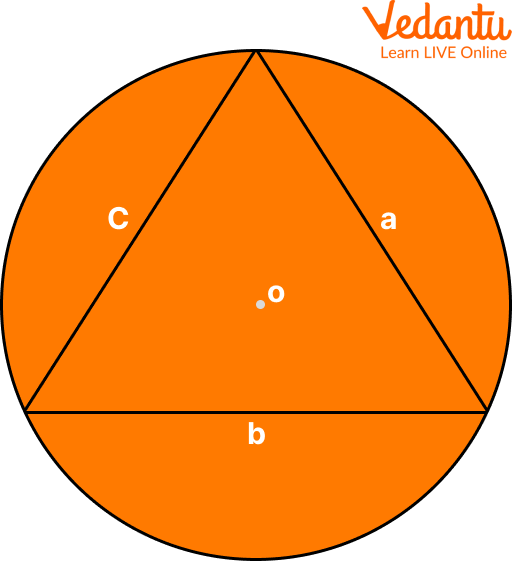Courses
Courses for Kids
Free study material
Free LIVE classes
MoreLIVE
Join Vedantu’s FREE Mastercalss

A triangle has three sides, two of those sides are called the base and the height. The perimeter of a triangle is the sum of the lengths of all the sides. If a triangle has three equal sides, then the perimeter equals three times the side length.The circumradius is the radius of a circle that will pass through all three vertices of a triangle. A triangle is surrounded by a single circle that is perpendicular to the base of the triangle. This is true for an equilateral triangle and any other type of triangle.

## What is Circumcircle?

A circumcircle is a circle that circumscribes any polygon and touches each polygon vertex. The radius of the circumcircle is the distance between the point of meeting of bisectors and the vertex. The circumcircle is drawn by taking the meeting point of the perpendicular bisector to the sides of the polygon as the centre.

The circumradius of a polygon or a triangle is the radius of the circle that circumscribes (surrounds) that polygon or triangle. It is the distance from the centre of the circumcircle to one of the vertices of the polygon or triangle. In the case of a triangle, the circumradius is also known as the circumcenter radius.

## How to Find Circumradius and What is the Formula?

Let that there is a circumcircle circumscribing a triangle with sides a, b and c with a circumcentre.$2 \mathrm{R}=\dfrac{a}{\operatorname{Sin} A}=\dfrac{b}{\operatorname{Sin} B}=\dfrac{c}{\sin C}$

Now, Area of triangle $\left(A_t\right)=\dfrac{1}{2} a b \operatorname{Sin} C$

$\operatorname{Sin} C=\dfrac{c}{2 R}$

Therefore, $\mathrm{A}_{\mathrm{t}}=\dfrac{1}{2} a b \dfrac{c}{2 R}$.

Now, $\mathrm{R}=\dfrac{a b c}{4 A}$

Hence, the required formula of circumradius of the triangle is $\mathrm{R}=\dfrac{a b c}{4 A}$.

## Solved Examples

1. What is the circumradius of a triangle with side lengths of 4, 5, and 6 units?

The circumradius of a triangle can be found using the formula:

$\mathrm{R}=\dfrac{a b c}{4 A}$.

where R is the circumradius, a, b, and c are the side lengths of the triangle, and A is the area of the triangle.

In this case, we have a triangle with side lengths of 4, 5, and 6 units.

To find the area of the triangle, we can use Heron's Formula:

$\text { Area } =\sqrt{s(s-a)(s-b)(s-c)}$

$\text { Area } =\text { area }$

$S =\text { semi-perimeter }$

$a=\text { length of side a }$

$b=\text { length of side } b$

$c=\text { length of side } c$

Where S is the semiperimeter of the triangle.

S = $\dfrac{(4+5+6)}{2} = 7.5$

$A=\sqrt{(7.5(7.5-4)(7.5-5)(7.5-6))}$

$A=\sqrt{(7.5(3.5)(2.5)(1.5))}$

$A=\sqrt{98.43}$

$=9.92$

So, we can plug the values into the formula:

$\mathrm{R}=\dfrac{a b c}{4 A}$

$= \dfrac{4\times 5\times 6}{4 \times 9.92} = 3.02$

Therefore, the circumradius of the triangle with side lengths of 4, 5, and 6 units is 3.02 units.

2. Triangle ΔABC is inscribed in a circle O, and side AC passes through the circle’s centre. Find the circle’s diameter.

We know that the triangle inscribed by a chord that passes through the centre of the circle is a right triangle.

Given, BC = 16 and AB = 12.

Hypotenuse theorem can be applied here,

$A C^{2}=A B^{2}+B C^{2}$

$=A C^{2}=12^{2}+16^{2}$

$=A C=\sqrt{12^{2}+16^{2}}$

$=A C=\sqrt{144+256}$

$= AC=\sqrt{400}$

$=A C=20$

The diameter of the circle is 20.

3. What is the area of a circle that is inscribed in a square of area 64 square units?

Inscribed circles in squares have a diameter equal to their sides when the circle is encircled. As a result, the radius of the inscribed circle is 4 units, while the diameter is 8 units.

The area of a circle of radius r units is $\mathrm{~A}=\pi \mathrm{r}^2$.

Substitute r=4 in the formula.

$\mathrm{~A}=\pi \mathrm{4}^2 = 16 \pi$≈50.24

Therefore, the area of the inscribed circle is about 50.24 square units.

## Practice Questions

1. The circumradius of the triangle, whose sides are 13,12 and 5, is

A) 15

B) 132

C) 6

Ans: 6 (Option C)

2. The in-radius and circumradius of a right-angled triangle are 3 cm and 12.5 cm, respectively. The area of the triangle is:

A) 84

B) 64

C) 94

Ans: 84 (Option A)

3. The in-radius and circumradius of a right-angled triangle are 2 cm and 10 cm, respectively. The area of the triangle is:

A) 4

B) 8

C) 13.5

Ans: 13.5 (Option C)

## Summary

The basic definition of circumradius in geometry is that it refers to the radius of a circle that passes through all three vertices of a triangle. In simple words, it is the radius of a circle that circumscribes the triangle. A triangle also has one circumscribed circle around it, which is perpendicular to its base. This is true for an equilateral triangle and any other type of triangle.

In conclusion, you can use the Law of Sines and the Law of Cosines to find the circumradius of a triangle. Do not forget to use the formula, $\mathrm{R}=\dfrac{a}{\operatorname{Sin} A}$ and you will be able to determine the value of A.

Last updated date: 02nd Oct 2023
Total views: 70.2k
Views today: 0.70k

## FAQs on Circumradius of a Triangle

1. What is the relationship between a triangle's circumradius and the inradius?

The circumradius and inradius of a triangle are related to each other through the circumradius-inradius inequality theorem, which states that the circumradius of a triangle is greater than or equal to twice the inradius.

2. What is the inradius of a triangle?

Inradius can be defined as the distance from the centre of a triangle to its incenter. The inradius is half the length of the altitude from the triangle's centre to its incenter and is a property of triangles.

3. How to find the radius of a circumscribed circle of an isosceles triangle?

An intersection of the perpendicular bisectors drawn to each side of the triangle defines the radius of a circumscribed circle in an isosceles triangle. An isosceles triangle has a perpendicular bisector at its base, equal to its height. In an isosceles triangle, the circumscribed circle's centre will be on the perpendicular bisector, which is also the height, median, and vertex angle bisector. The formula used is is given as follows: $R=\dfrac{a^{2}}{ \sqrt{4 a^{2}-b^{2}}}$.